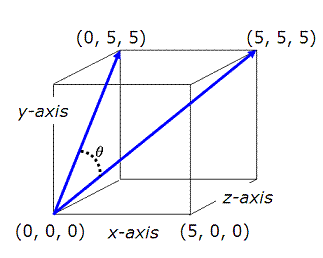# Rotation of two vectors

Given two three-dimensional unit vectors A and B, calculate the rotation angle (including the order) from A to B.

Hi, Koly,

If I understood right, you need the angle between the vectors at the origin:The angle θ can be simply calculated with: θ = acos[ (u.v) / (|u||v|) ]. acos() is the arc-cosine function. u.v is the dot product between the two vectors. |u||v| is the product between the lengths of both vectors.

If this is not what you want, you need to state your problem with further details.

cheers,

Paulo